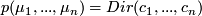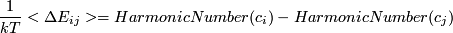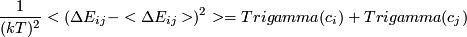# Page EnergyErrorEstimation

We propose a new approximatiom to analytically predict errors in energy differences. The underlying probability of transitions between states are given by a dirichlet distribution.

#### Error in the equilibrium distribution

We use a method, developed mainly by Nina Singhal / Vijay Pande (s. paper...), where one can analytically predict a linear approximation to the error in eigenvectors and eigenvalues of the transition matrix. Since the equilibrium distribution can be expressed in terms of the first left eigenvectors we can use this scheme to compute the covariance matrix for the equilibrium distribution along with the mean value to first order.

Assuming, that this is sufficient, we could use the given gauss distribution for the entries to compute the mean values and variances for all energy differences. This would be given by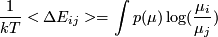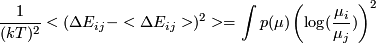We have now several possibilities

We can use the Gauss Distribution from Ninas method and use this in the functions above. This works, but only numerically, since there exists no analytical solution, especially not in the couplet case, where the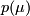cannot be seperated into single parts for each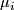so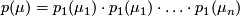In this case we can simplify the mean value to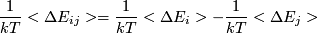with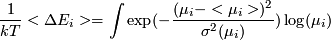for this expression there exists no analytical expression, but it still is well defined. I did not investigate in the case, where the probability cannot be seperated. It might turn out, that in some rare cases the energy might not even be well-defined. Aside from the numerical solution or even sampling techniques we want to take another approach:

We know that each, they sum up to one and that the probabilities at the borders vanish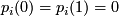. Furthermore the function is continous and we will assume, that the mean is known, namely given by the linear translation of the method of Nina Singhal. The last point is, that we know for lots of statistics, that it will converge to a MVN Distribution.

It is obvious, that the assumed Gaussian does not fulfill these simple requirements and in fact, there is no reason to stick to the Gauss function, except, that around the mean (actually it should be the maximum) it is Gaussshaped to first order (so is any monomodal distribution) and for the central limit theorem. We do not deal with high statistics and thus we have to idea, what the real distribution will look like. We could assume, that there is a distinct maximum, but that is not save. Since the Gaussfunction cannot be used to find an analytical solution anyway, why not use another distribution, that catches all the properties. Since we started with Dirichlet Distribution, why not stick to this? It fulfills all requirements! That does not mean, that the solution is correct, but it might be a better try, than using a simple Gaussian (even, if the results will not be that different as we will see)

We will assume, that we can represent the equilibrium distribution also by a Dirichlet distribution. But what about the analytical expression for the mean / variance of the energy differences? Here we are also lucky, there exist reasonable simple expresion, that can be computed fast to high accuracy.

One imminent difference is a problem in this. The MVN has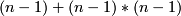independant parameters. The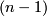from the-mean values, that sum up to one and the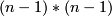vom the CoVariance-Matrix, that has rowsum of one to ensure, that the values keep the sum of one or in other words, one eigenvalue is zero. The Dirichlet Distribution in contrast needs onlyParameters.

One first idea we used is to keep all the mean values. By that we can fix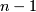Parameters of the Dirichlet Distribution. That only misses the overall scaling factor that influences the overall error and sharpness of the function. The problem in this is, that the variances in various directions are all very similar, that might cause a problem and we will investigate further in this...

For the case of 2 states the results are similar, but the Dirichlet Approximatin is closer to the exact result, but here we do not have the problem with the overdetermined parameters.

If we have too much problem with this states, it might be easier to represent each single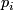by a 2state Dirichlet Funtion, but this would loose the sum of one contraint.

Finally we present the solutions to the Dirichlet Case